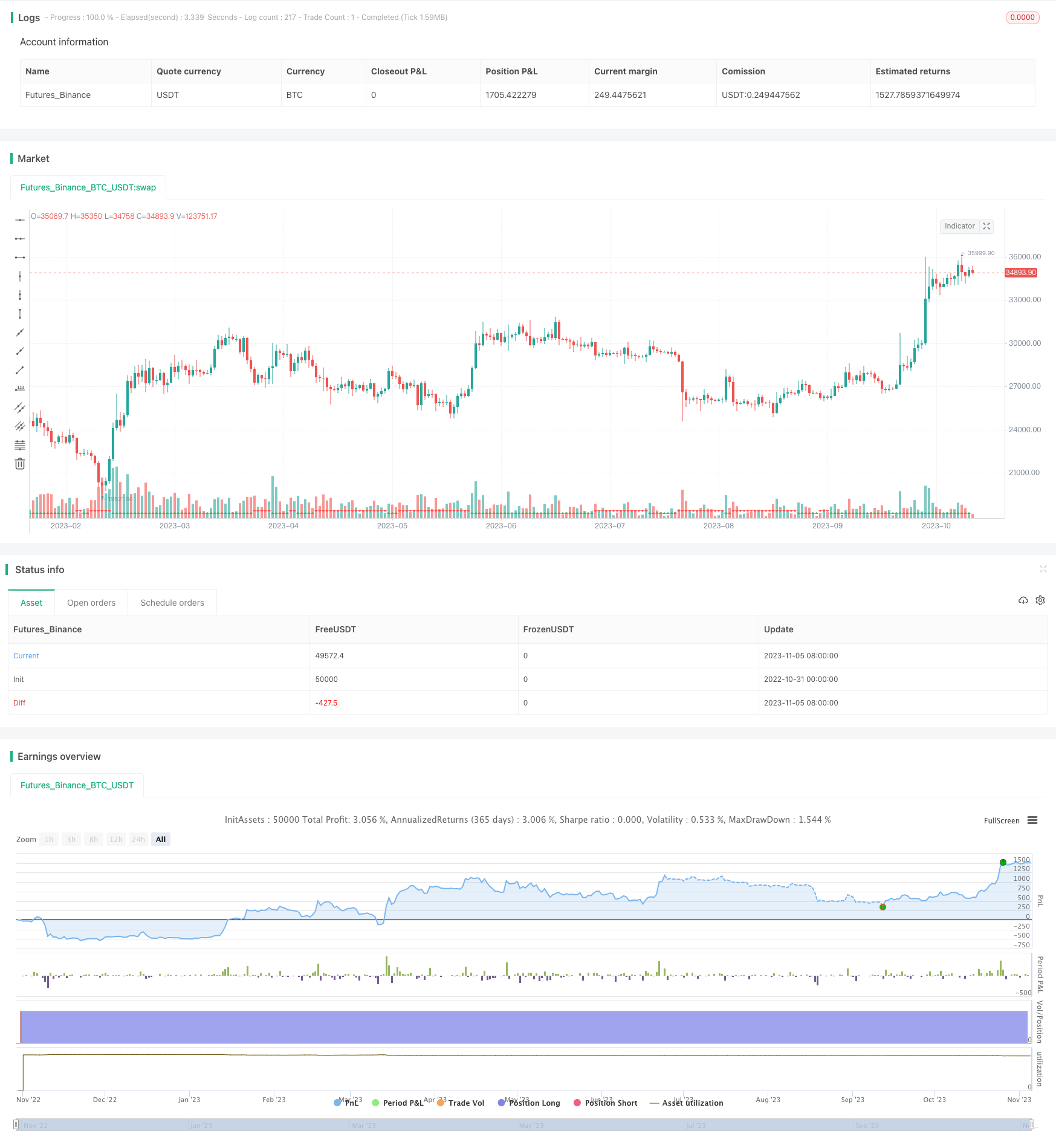# Dual Timeframe DI Trend Following Strategy

Author: ChaoZhang, Date: 2023-11-07 16:31:07
Tags:## Overview

This strategy uses Average Directional Index (DI+) and Negative Directional Index (DI-) on two timeframes to determine the trend direction for long and short trades. When DI+ is higher than DI- on both larger and smaller timeframes, it indicates an upward trend and a long signal is triggered. When DI- is higher than DI+ on both frames, it indicates a downward trend and a short signal is triggered.

## How it Works

The strategy is based on several principles:

1. Calculate DI+ and DI-. Get DI+ and DI- by using high, close and low prices.

2. Compare DI+ and DI- on two timeframes. Calculate DI+ and DI- respectively on the main chart timeframe (e.g. 1 hour) and a larger timeframe (e.g. daily). Compare the values between the two timeframes.

3. Determine trend direction. When DI+ is greater than DI- on both larger and smaller timeframes, it indicates an upward trend. When DI- is greater than DI+ on both frames, it indicates a downward trend.

4. Trigger trading signals. DI+>DI- on both frames gives long signal. DI->DI+ on both frames gives short signal.

5. Set stop loss. Use ATR to calculate dynamic stop loss for trend following.

6. Exit conditions. Exit when stop loss is hit or price reverses.

The strategy has the following advantages:

1. Using dual timeframe DI filters out some false breakouts.

2. ATR trailing stop maximizes profit protection and avoids stops being too tight.

3. Timely stop loss controls loss on single trades.

4. Trading with the trend allows continuously catching trends.

5. Simple and clear rules, easy to implement for live trading.

## Risks and Solutions

There are also several risks:

1. DI has lagging effect, may miss entry timing. Can optimize parameters or add other indicators.

2. Dual timeframe may have divergence between larger and smaller TF. Add more timeframe validation.

3. Stop loss too aggressive may cause over-trading. Loosen ATR multiplier.

5. Parameter optimization relies on historical data and may be overfitted. Evaluate parameter robustness prudently.

## Optimization Directions

The strategy can be improved in the following aspects:

1. Optimize DI calculation parameters for best parameter set.

2. Add other indicator filters to improve signal accuracy, e.g. MACD, KDJ etc.

3. Enhance stop loss strategy to adapt more market conditions, such as trailing stop or pending orders.

5. Test parameter robustness on different products to improve adaptiveness.

6. Introduce machine learning to train model on historical data.

## Conclusion

In summary, this is a typical trend following strategy that uses DI to determine trend direction and set stop loss to lock in profits along the trend. The advantage lies in its clear logic and ease of implementation for live trading. There are also rooms for improvement via parameter optimization, adding filters etc. With further optimization and robustness test, it can become a very practical trend following strategy.

```/*backtest
start: 2022-10-31 00:00:00
end: 2023-11-06 00:00:00
period: 1d
basePeriod: 1h
exchanges: [{"eid":"Futures_Binance","currency":"BTC_USDT"}]
*/

// This source code is subject to the terms of the Mozilla Public License 2.0 at https://mozilla.org/MPL/2.0/

//@version=5
strategy("DI+/- multi TF Strat [KL]", overlay=true, pyramiding=1, initial_capital=1000000000, default_qty_type=strategy.percent_of_equity, default_qty_value=5)
var string GROUP_SL       = "Stop loss"
var string GROUP_ORDER    = "Order size"
var string GROUP_TP       = "Profit taking"
var string GROUP_HORIZON  = "Time horizon of backtests"
var string GROUP_IND      = "Directional IndicatorDI+ DI-"

adx_len = input(14, group=GROUP_IND, tooltip="Typically 14")
tf1 = input.timeframe("", title="DI +/- in Timeframe 1", group=GROUP_IND, tooltip="Main: DI+ > DI-")
tf2 = input.timeframe("1D", title="DI +/- in Timeframe 2", group=GROUP_IND, tooltip="Confirmation: DI+ > DI-")
// adx_thres = input(20, group=GROUP_IND)   //threshold not used in this strategy

// (high, close, mid) -> [plus_DM, minus_DM]
// Based on TradingView user BeikabuOyaji's implementation
_tr = math.max(math.max(_high - _low, math.abs(_high - nz(_close))), math.abs(_low - nz(_close)))
smooth_tr = 0.0
smooth_tr := nz(smooth_tr) - nz(smooth_tr) / adx_len + _tr

smooth_directional_mov_plus = 0.0
smooth_directional_mov_plus := nz(smooth_directional_mov_plus) - nz(smooth_directional_mov_plus) / adx_len + (_high - nz(_high) > nz(_low) - _low ? math.max(_high - nz(_high), 0) : 0)

smooth_directional_mov_minus = 0.0
smooth_directional_mov_minus := nz(smooth_directional_mov_minus) - nz(smooth_directional_mov_minus) / adx_len + (nz(_low) - _low > _high - nz(_high) ? math.max(nz(_low) - _low, 0) : 0)

plus_DM = smooth_directional_mov_plus / smooth_tr * 100
minus_DM = smooth_directional_mov_minus / smooth_tr * 100
// DX = math.abs(plus_DM - minus_DM) / (plus_DM + minus_DM) * 100   // DX not used in this strategy
[plus_DM, minus_DM]

// DI +/- from timeframes 1 and 2
[plus_DM_tf1, minus_DM_tf1] = get_ADX(request.security(syminfo.tickerid, tf1, high), request.security(syminfo.tickerid, tf1, close),request.security(syminfo.tickerid, tf1, low))
[plus_DM_tf2, minus_DM_tf2] = get_ADX(request.security(syminfo.tickerid, tf2, high),request.security(syminfo.tickerid, tf2, close),request.security(syminfo.tickerid, tf2, low))
// } end of block: ADX Indicator

var string ENUM_LONG      = "LONG"
var string LONG_MSG_EXIT  = input.string("Long closed", title="Alert MSG for closing (Long position)", group=GROUP_ALERT)
backtest_timeframe_start = input(defval=timestamp("01 Apr 2020 13:30 +0000"), title="Backtest Start Time", group=GROUP_HORIZON)
within_timeframe         = true

// Signals for entry
_uptrend_confirmed = plus_DM_tf1 > minus_DM_tf1 and plus_DM_tf2 > minus_DM_tf2
entry_signal_long = _uptrend_confirmed

plotshape(_uptrend_confirmed, style=shape.triangleup, location=location.bottom, color=color.green)
plotshape(not _uptrend_confirmed, style=shape.triangledown, location=location.bottom, color=color.red)

// Trailing stop loss ("TSL") {
tsl_multi                 = input.float(2.0, title="ATR Multiplier for trailing stoploss", group=GROUP_SL)
SL_buffer                 = ta.atr(input.int(14, title="Length of ATR for trailing stoploss", group=GROUP_SL)) * tsl_multi
TSL_source_long           = low
var stop_loss_price_long  = float(0)
var pos_opened_long       = false

stop_loss_price_long := pos_opened_long ? math.max(stop_loss_price_long, TSL_source_long - SL_buffer) : TSL_source_long - SL_buffer

// MAIN: {
if pos_opened_long and TSL_source_long <= stop_loss_price_long
pos_opened_long := false
strategy.close(ENUM_LONG, comment=close < strategy.position_avg_price ? "stop loss" : "take profit")

// (2) Update the stoploss to latest trailing amt.
if pos_opened_long
strategy.exit(ENUM_LONG, stop=stop_loss_price_long, comment="SL")

// (3) INITIAL ENTRY:
if within_timeframe and entry_signal_long
pos_opened_long := true
strategy.entry(ENUM_LONG, strategy.long, comment="long")

// Plotting:
TSL_transp_long = pos_opened_long and within_timeframe ? 0 : 100
plot(stop_loss_price_long, color=color.new(color.green, TSL_transp_long))

// CLEAN UP: Setting variables back to default values once no longer in use
if ta.change(strategy.position_size) and strategy.position_size == 0
pos_opened_long := false

if not pos_opened_long
stop_loss_price_long := float(0)

// } end of MAIN block

```

More